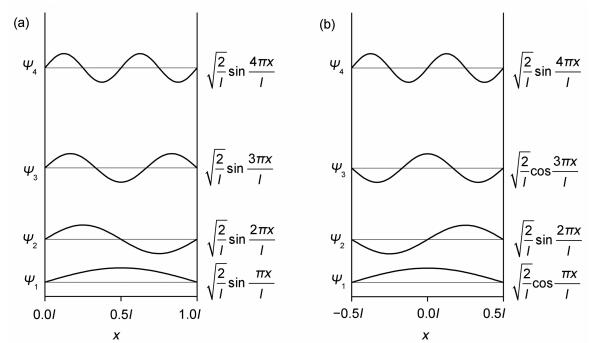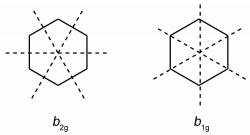## Boundary Conditions and Wave Functions of the Particle in a Box

,,,Abstract

In this paper, we discuss the particle in a one-dimensional box and the particle on a ring. The influence of boundary conditions on levels and wave functions has also been analyzed for these two typical systems.

Keywords： Structural chemistry ; Particle in a box ; Boundary conditions

CHEN Lan, SUN Hong-Wei, LAI Cheng-Ming. Boundary Conditions and Wave Functions of the Particle in a Box. University Chemistry[J], 2017, 32(5): 77-80 doi:10.3866/PKU.DXHX201608030

## 1 一维自由运动的粒子

$-\frac{{{\hbar ^2}}}{{2m}}\frac{{{{\text{d}}^2}\psi \left( x \right)}}{{{\text{d}}{x^2}}} = E\psi \left( x \right)$

${k^2} = \frac{{2mE}}{{{\hbar ^2}}} = \frac{{{p^2}}}{{{\hbar ^2}}}$,其复数形式的通解为ψ(x) = c1exp (i| p|x/ħ) + c2exp (－i| p|x/ħ)，实数形式的通解为ψ(x) = c1 cos (| p|x/ħ) + c2 sin (| p|x/ħ)。通常采用形式最为简单的复数特解exp (±i| p|x/ħ)或实数特解cos (| p|x/ħ)和sin (| p|x/ħ)这两对线性独立的解表示粒子的状态，复数解和实数解描述不同的运动方式。

${\mathit{\Psi }}\left( {x, t} \right) = A\exp \left[{i\left( {|p|x-Et} \right)/\hbar } \right]$

${\mathit{\Psi }}\left( {x, t} \right) = A\exp \left[{i2{\pi }\left( {x/\lambda-vt} \right)} \right]$

$\begin{gathered} {{\hat p}_x}\left[{A\exp \left( { + i|p|x/\hbar } \right)} \right] = - i\hbar \frac{{\text{d}}}{{{\text{d}}x}}\left[{A\exp \left( { + i|p|x/\hbar } \right)} \right] = + |p|\left[{A\exp \left( { + i|p|x/\hbar } \right)} \right] \hfill \\ {{\hat p}_x}\left[{A\exp \left( {-i|p|x/\hbar } \right)} \right] = - i\hbar \frac{{\text{d}}}{{{\text{d}}x}}\left[{A\exp \left( {-i|p|x/\hbar } \right)} \right] = - |p|\left[{A\exp \left( {-i|p|x/\hbar } \right)} \right] \hfill \\ \end{gathered}$

$\begin{gathered} \cos (|p|x/\hbar ) = \left[{\exp \left( {i|p|x/\hbar } \right) + \exp \left( {-i|p|x/\hbar } \right)} \right]/2 \hfill \\ \sin (|p|x/\hbar ) = \left[{\exp \left( {i|p|x/\hbar } \right)-\exp \left( {-i|p|x/\hbar } \right)} \right]/2i \hfill \\ \end{gathered}$

## 2 一维势箱中的粒子

### 图1(a)势箱范围0 ≤ xl；(b)势箱范围－l/2 ≤ xl/2

## 3 圆环上的粒子

### 图2### 图3## 参考文献 原文顺序 文献年度倒序 文中引用次数倒序 被引期刊影响因子

/

 〈〉